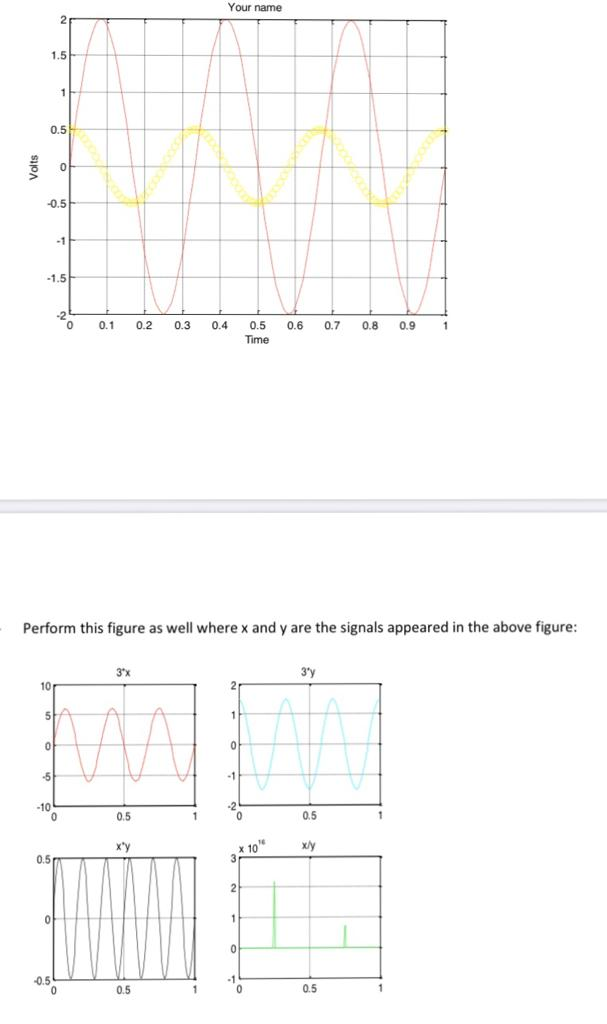# how to write a code to get these figures ??

8 views (last 30 days)
mike john on 21 Apr 2020
Commented: Rena Berman on 1 Jun 2020Rena Berman on 1 Jun 2020

KSSV on 21 Apr 2020
Edited: KSSV on 21 Apr 2020
th = linspace(0,2*pi) ;
x1 = sin(th) ;
x2 = cos(th) ;
figure(1)
plot(th,x1)
figure(2)
plot(th,x1,'r') ;
hold on
plot(th,x2,'b') ;
figure(3)
subplot(121)
plot(th,x1)
subplot(122)
plot(th,x2)

BALAJI KARTHEEK on 21 Apr 2020
this code will help you out, but i am not sure about the graph of fourth figure but x/y yields to tan graph that is what i am getting since x is sin function and y is cos function
clc
clear all
close all
a1=2;
a2=0.5;
t=0:0.01:1;
T=0.33;
y1=a1*sin(2*pi*t/T);
y2=a2*cos(2*pi*t/T);
figure
plot(t,y1)
hold on
plot(t,y2,'o')
grid on
ylabel('Time')
xlabel('Volts')
figure
subplot(2,2,1)
plot(t,3*y1)
title('3*x')
subplot(2,2,2)
plot(t,3*y2)
title('3*y')
subplot(2,2,3)
plot(t,y2.*y1)
title('x*y')
subplot(2,2,4)
plot(t,y1./y2)
title('x/y')